## Homework 5

1. At, a 1D harmonic oscillator is in a linear combination of the energy eigenstates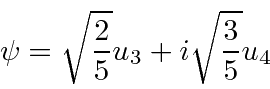Find the expected value ofas a function of time using operator methods.

2. Evaluate the uncertainty'' infor the 1D HO ground statewhere. Similarly, evaluate the uncertainty infor the ground state. What is the product? Now do the same for the first excited state. What is the productfor this state?

3. An operator is Unitary if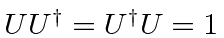. Prove that a unitary operator preserves inner products, that is. Show that if the states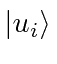are orthonormal, that the statesare also orthonormal. Show that if the statesform a complete set, then the statesalso form a complete set.

4. Show at if an operatoris hermitian, then the operatoris unitary.

5. Calculateand.

6. Calculate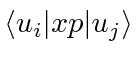by direct calculation. Now calculate the same thing using.

7. Ifis a polynomial in the operator, show that. As a result of this, note that since any energy eigenstate can be written as a series of raising operators times the ground state, we can representby.

8. Ata particle is in the one dimensional Harmonic Oscillator state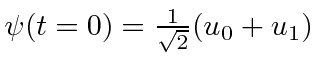.
• Compute the expected value ofas a function of time usingandin the Schrodinger picture.
• Now do the same in the Heisenberg picture.

Jim Branson 2013-04-22